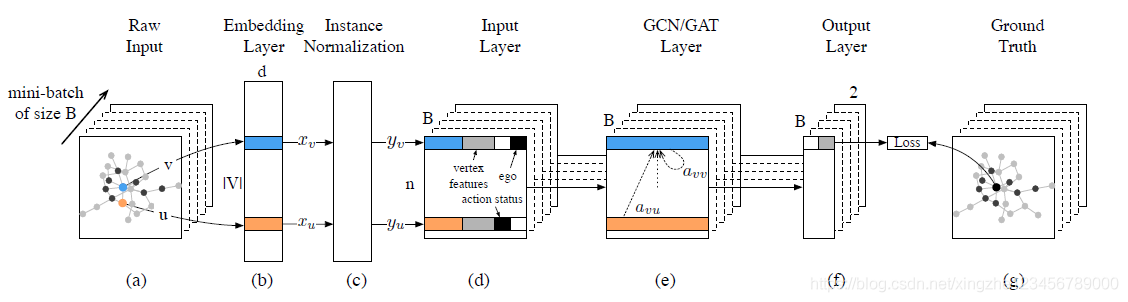KDD18一篇将GNN应用于社交网络中用户影响力预测任务的论文

解决的问题

问题定义

• 邻域：给定图$G=(V,E)$，顶点$v$的邻域定义为$N_v^r=\{u:d(u,v)\le r\}$，是一个顶点集合，不包含顶点$v$自身
• 中心网络：由邻域中的顶点及边所组成的网络，以$G_v^r$表示
• 用户行为：以$s_v^t$表示，用户对应于图中的顶点，对于一个时刻$t$，如果顶点$v$有产生动作，例如转发、引用等，则$s_v^t=1$

做法及创新

数据预处理